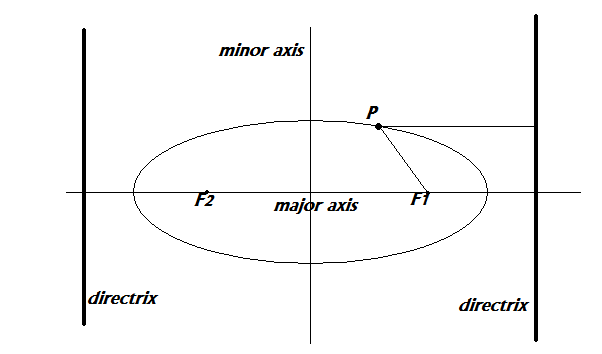### The Ellipse- Tutorial, Solved Problems, MCQ Quiz/Worksheet- Eccentricity, Focus, Major/Minor Axis, Chords, Tangents,Normals

Target Audience: High School Students, College Freshmen and Sophomores, Class 11/12 Students in India preparing for ISC/CBSE and Entrance Examinations like the IIT-JEE
Main or Advanced/AIEEE, and anyone else who needs this Tutorial as a reference!

After reading this tutorial you might want to check out some of our other Mathematics Quizzes as well.
 Quizzes on ProgressionsMCQ #1: Arithmetic Progression MCQ #2: Geometric ProgressionMCQ #3 : More on Geometric Progressions.MCQ #4 : Harmonic Progressions. MCQ #5: More on Harmonic ProgressionMCQ #6: Mixed ProgressionsComplex NumbersMCQ #1MCQ #2: More on Complex NumbersQuadratic EquationsMCQ Quadratic EquationsQuadratic In-equationsMCQ Quadratic In-equations Coordinate Geometry - Straight LinesMCQ #1: Cartesian Planes, Straight Line BasicsMCQ #2 on Straight LinesMCQ #3 on Straight LinesMCQ #4 on Straight LinesCircles1 MCQ #1 on Circles. 2 MCQ #2 on Circles. 3 MCQ #3 on Circles. Conic Sections- Parabola, Hyperbola, Ellipse1 MCQ- The Basics of Conic Sections2 MCQ on Parabola..3 MCQ on Hyperbola4 MCQ on Ellipses. ProbabilityMCQ #1 on Basic ProbabilityMCQ #2: More Challenging Problems on ProbabilityMCQ #3- Conditional Probability and Bayes Theorem

## The Ellipse: Equation of an Ellipse, Eccentricity, Focus, Major and Minor Axis, Chords and Latus Rectum:

• The ellipse is the locus of a point in a plane such that the ratio of distance of that point from a fixed point, focus, to the ratio of the distance of that point from a fixed line, the directrix, is constant and is always less than one.
• The ratio is called eccentricity, denoted by e.
• The equation ax2+2hxy+by2+2gx+2fy+c=0 denotes an ellipse when abc+2fgh-af2-bg2-ch2≠0 and h2-ab<0.
• Every ellipse has two axis, major and minor. The major axis is perpendicular to directrix and passes through the focus.
• Also, there are two ‘focus’ in an ellipse, and hence two ‘directrix’, one corresponding to each. Every point’s distance from each focus to the point’s distance from the corresponding directrix is in a constant ratio e.
• The minor axis is parallel to the directrix and bisects the line joining the two foci. The point where major and minor axis meet is called the center of ellipse.• Chord: A line segment whose end points lie on the ellipse.
• Focal Chord: A chord passing through ellipse.
• Double Ordinate: A chord perpendicular to the major axis.
• Latus Rectum: A double ordinate passing through focus.
• The equation of ellipse whose axis are parallel to the co-ordinate axes, and whose centre is origin is x2a2+y2b2=1.

## Important Formulas and Equations

a>b a<b
Coordinates of Center(0,0)(0,0)
Coordinates of Vertices(a,0) and (-a,0)(0,-b) and (0,b)
Coordinates of Foci(ae,0) and (-ae,0)(0,be) and (0,-be)
Length of Major Axis2a2b
Length of Minor Axis2b2a
Equation of Major Axisy=0x=0
Equation of Minor Axisx=0y=0
Equation of Directricesx=a/e and x=-a/ey=b/e and y=-b/e
Eccentricity
e=1-b
2/a2

## e=1-a2/b2

Length of Latus Rectum 2b2/a  2a2/b
Focal Distance of a Pointa±exb±ey
Diagram

## Parametric Form, Tangents, Normals, Chords and Diameter of an Ellipse

Note :  The results are for the standard form of ellipse!

### Parametric Form

Parametric  form of ellipse is (acosθ,bsinθ)

### Tangent

xx1/a2+yy1/b2=1 , at point (x1,y1) y=mx±a2m2+b2, the point of contact being (±a2ma2m2+b2,±b2a2m2+b2)
or (xcosθ)a+(ysinθ) b=1, the point of contact being (acosθ,bsinθ)
From an external point, two tangents can be drawn to the ellipse.

### Normals

a2x-x1x1=b2y-y1y1, point of contact being x1,y1, upon simplification
a2xy1-b2yx1-a2-b2x1y1=0

axcosθ+bysinθ=a2-b2, the point of contact being (acosθ,bsinθ)
From a fixed pint, four normals can be drawn to the ellipse.

### Chord

Equation of chord joining (acosα,bsinα) and (acosβ,bsinβ) is
xacosα+β2+y2sinα+β2=cosα-β2
*The locus of the point of intersection of two perpendicular tangents to an ellipse is a circle known as the director circle.
*Auxiliary circle of an ellipse is a circle which is described on the major axis of an ellipse as its diameter.
*Diameter.
The locus of mid points of a system of parallel chords is called the diameter.

*Conjugate Diameters.
Two diameters are conjugate diameters which bisects chords parallel to each other.
Condition turns out to be mm
1=-b2/a2
The only pair of conjugate diameters that are perpendicular to each other and does not satisfy the above condition is the major and minor axis of ellipse.
If two conjugate diameters are equal, then they are called equi-conjugate diameters.
.

#### Here are some of the problems solved in this tutorial :

Q: Find the equation of ellipse with focus at (1,1), eccentrity ½ and directrix x-y+3=0. Also, find the equation of its major axis.

Q: Find lengths of major and minor axes, coordinates of foci and vertices, and the eccentrity of x2+4y2-2x=0.

Q:  A man running a race course notes that the sum of distances from the two flag posts is always 10 metres and the distance between the flag posts is 8 metres. Find the equation of the path traced by him.

Q: Find the locus of the point of intersection of the tangents which meet at right angles.

Q:  Prove that the product of the lengths of perpendiculars drawn from foci to any tangent is b2. (a>b)

Q: If the normals at the end of a latus rectum passes through the extremity of a minor axis, prove that e4+e2=1.

Q: Find the chord of contact wrt point (x1,y1).

Q: Find the equation of a pair of tangents drawn from P(x1,y1)

Q: Prove that the common tangent of the circle x2+y2=4 and x27+y23=1 is inclined to major axis at an angle of 30 degrees.

Q: Find the equation of a chord of the ellipse whose mid point is (x1,y1)